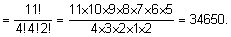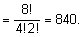In how many of the the distinct permutations of the letters in MIS | FiloClass 11

Math

Algebra

Permutations and Combinations585

In how many of the the distinct permutations of the letters in do the four 's not come together?

Solution:

The word MISSISSIPPI has one M, four I’s, four S’s, two P’s and a total of 11 letters.

The number of all type of arrangements possible with the given alphabetsLet us first find the case when all the I’s together and so take it as one packet or unit. So now we have one M, one unit of four I’s, four S’s, two P’s and a total of 8 units.

Therefore the number of arrangements possible when all the I’s is togetherHence, the distinct permutations of the letters of the word MISSISSIPPI when four I’s do not come together = 34650 – 840 = 33810.585Connecting you to a tutor in 60 seconds.